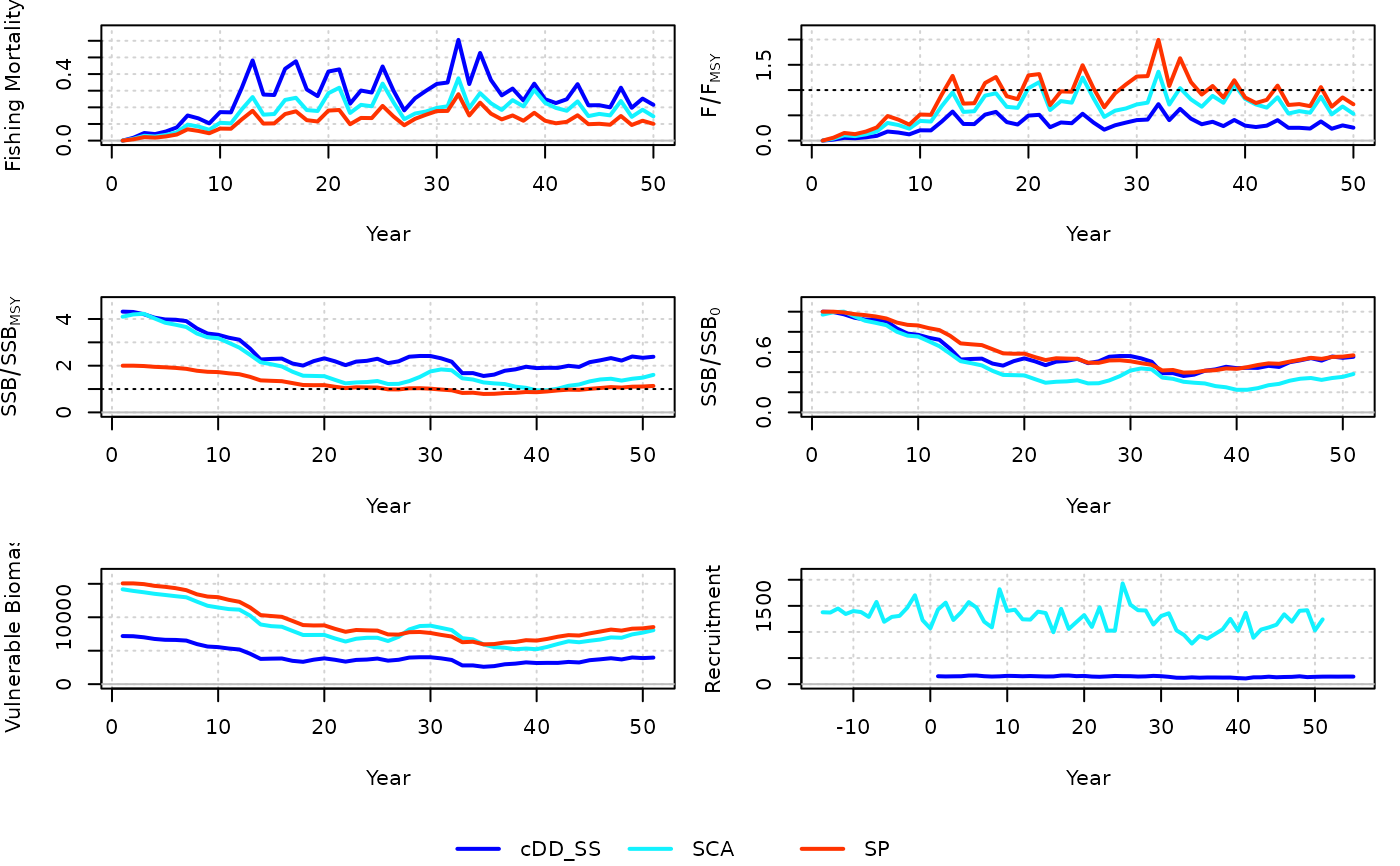Plot biomass, recruitment, and fishing mortality time series from several . This function can be used to compare outputs among different assessment models from the same Data object.

compare_models(..., label = NULL, color = NULL)

## Arguments

...

Objects of class Assessment.

label

A character vector of the models for the legend.

color

A vector of colors for each assessment model.

## Value

A set of figures of biomass, recruitment, and fishing mortality estimates among the models.

Q. Huynh

## Examples

res <- cDD_SS(x = 3, Data = MSEtool::SimulatedData)
res2 <- SCA(x = 3, Data = MSEtool::SimulatedData)
res3 <- SP(x = 3, Data = MSEtool::SimulatedData)

compare_models(res, res2, res3)